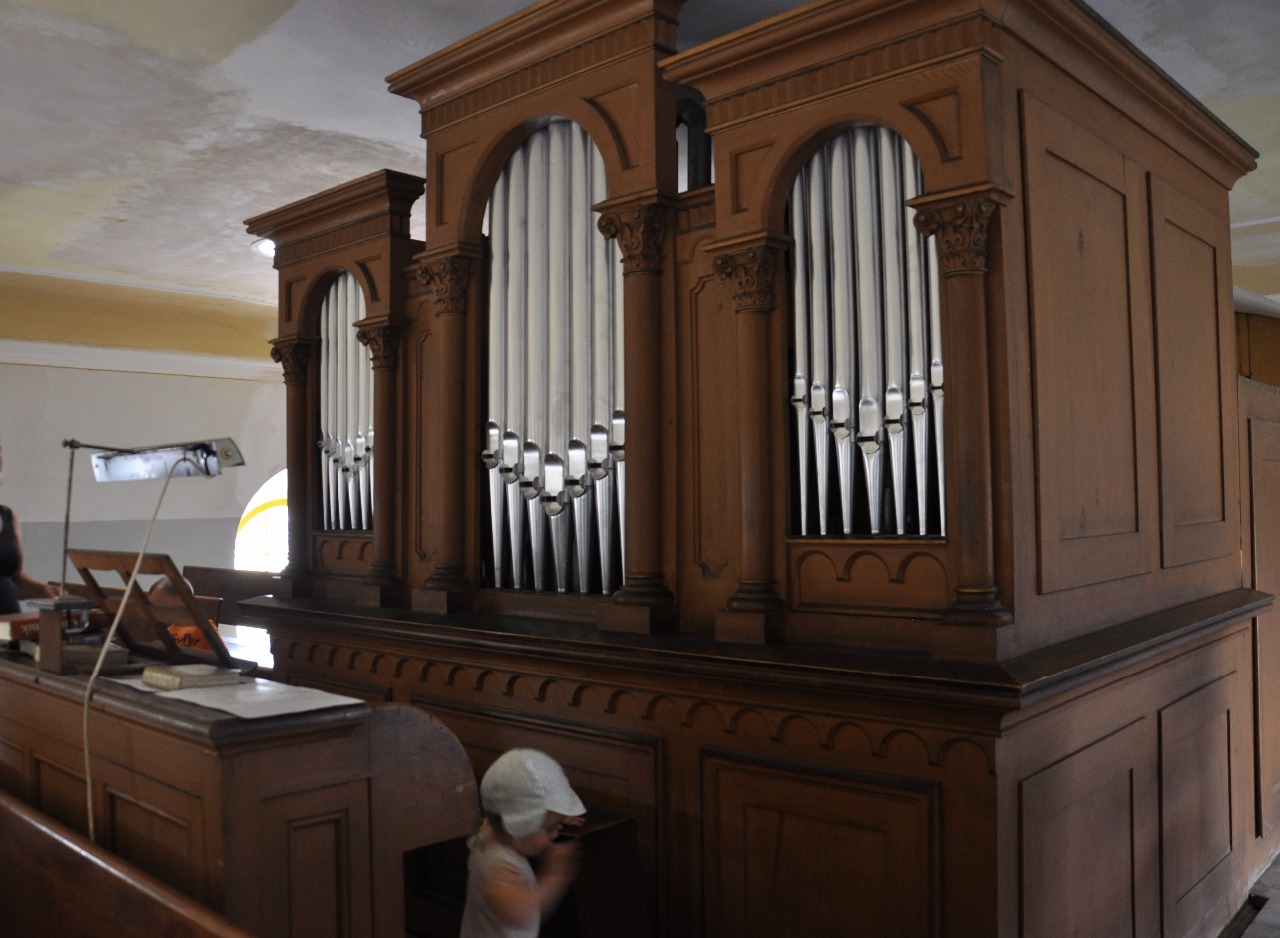# LOGARITHMIC PRESENTATION OF MUSICAL INTERVAL IN BACH ́S TUNING

LOGARITHMIC PRESENTATION OF MUSICAL INTERVAL IN BACH ́S TUNING

Karel Irmann
Slunecna 824, Horovice CZ 26801
irmannkarel@seznam.cz

Abstract: Musical interval is a distance between two tones which can be defined by the diference of decimal logarithms of frequence this two tones. Presented project explicitly numericaly defines how Bach ́s tuning chase size of the interval of the major third.

Bach ́s tuning C – komplex J.S. Bach (1685 – 1750)

Ges4 - Bb4 = 0,10235 Des4 – F4 = 0,10235 As4 – C5 = 0,10235

Es4 - G4 = 0,10184 Bb4 - D5 = 0,10034 F4 - A4 = 0,09884

C4 - E4 = 0,09708 G4 - B4 = 0,09683 D4 - Fis4 = 0,09835

A4 - Cis4 = 0,09984 E4 - Gis4 = 0,10159 B3 - Dis4 = 0,10234

5/4 = 0,09691 81/64 = 0,10230

1 Elaboration
The Bach ́s irregularly tempered tuning was documented by the composition cycle „Das wohltemperierte Klavier“.
In the Table 1., the division of the musical extent in octaves is shown. The tone frequencies are in Hz, corresponding are indicated to the frequencies. The size of the octave interval of 0,30103 is defined by the diference of the decadic logarithms of the frequencies indicated.
In the Table 2., one octave A4 - A5 (a1 – a2) is dividend in twelwe halftone steps corresponding to the Bach ́s tuning. The deviations from the regularly tempered tuning in cents were obtained from the Dr. Kelletat ́s data for the company „ Wandel und Goltermann“.
In the Table 3., the major thirds are shown in the semence of the quint-fourth circle. The Cdur major thirds areindicated appr. In the centre of the table. The values of the diference of the logarithms indicated aproach to the value of the natural major third 5/4 = 0,09691. At the beginning and end of the table, the values aproach to the major third 81/64 = 0,10230.

This finding corresponds to the acoustic evaluation of the Bach ́s tuning mentioned in specialized musical literature.